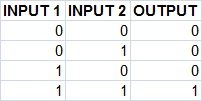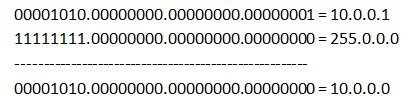An IP address is divided into two parts: network and host parts. For example, an IP class A address consists of 8 bits identifying the network and 24 bits identifying the host. This is because the default subnet mask for a class A IP address is 8 bits long. (or, written in dotted decimal notation, 255.0.0.0). What does it mean? Well, like an IP address, a subnet mask also consists of 32 bits. Computers use it to determine the network part and the host part of an address. The 1s in the subnet mask represent a network part, the 0s a host part.

Computers works only with bits. The math used to determine a network range is binary AND.Let’s say that we have the IP address of 10.0.0.1 with the default subnet mask of 8 bits (255.0.0.0).
First, we need to convert the IP address to binary: